# Current Electricity Current Electricity n What is it

• Slides: 12Current Electricity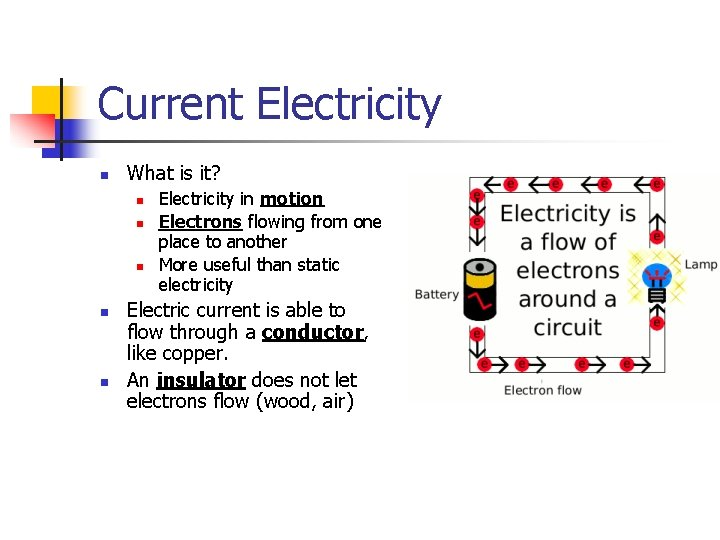Current Electricity n What is it? n n n Electricity in motion Electrons flowing from one place to another More useful than static electricity Electric current is able to flow through a conductor, like copper. An insulator does not let electrons flow (wood, air)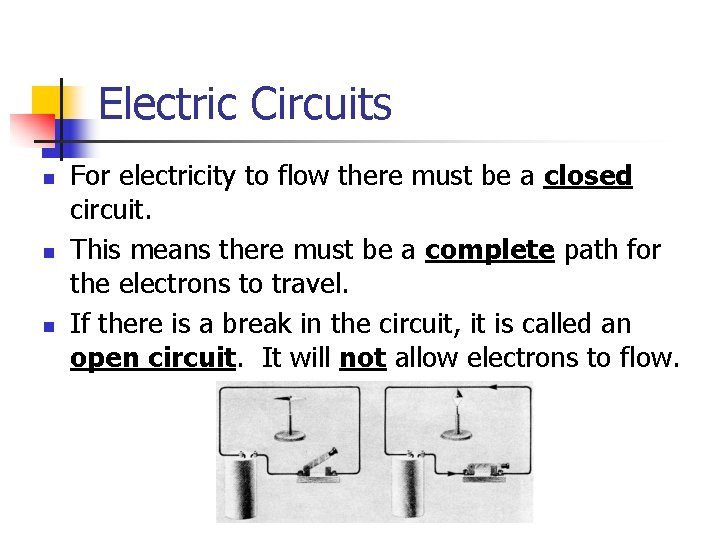Electric Circuits n n n For electricity to flow there must be a closed circuit. This means there must be a complete path for the electrons to travel. If there is a break in the circuit, it is called an open circuit. It will not allow electrons to flow.Electric Circuits n n An electrical circuit must have an energy source, such as a battery or a power supply that pushes the electrons around the circuit. A 1. 5 V dry cell that you would use at home is an example of a device that supplies 1. 5 volts of force to push electrons around the circuit. A 12 volt car battery is very similar, it uses six 2 V cells connected together to produce 12 volts of force.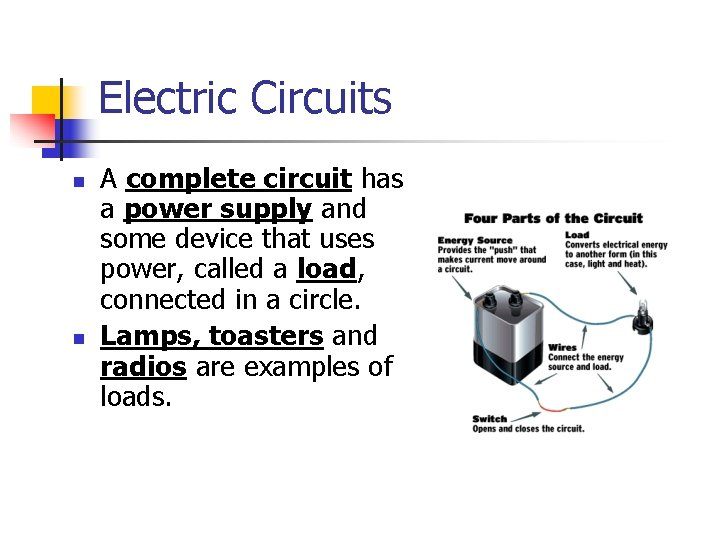Electric Circuits n n A complete circuit has a power supply and some device that uses power, called a load, connected in a circle. Lamps, toasters and radios are examples of loads.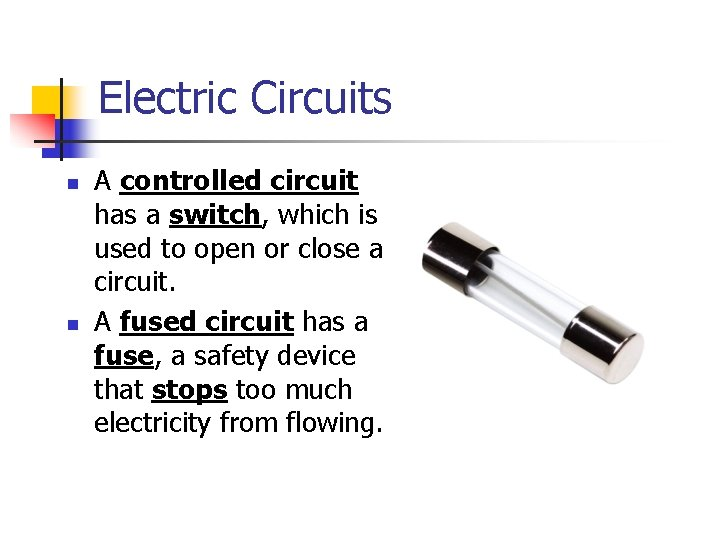Electric Circuits n n A controlled circuit has a switch, which is used to open or close a circuit. A fused circuit has a fuse, a safety device that stops too much electricity from flowing.Electric Circuits n n n A short circuit has a path that lets electrons flow, but it has no load. It is very dangerous. Electrons in a circuit flow out of the negative terminal and back to the positive terminal. This movement of electrons is called current.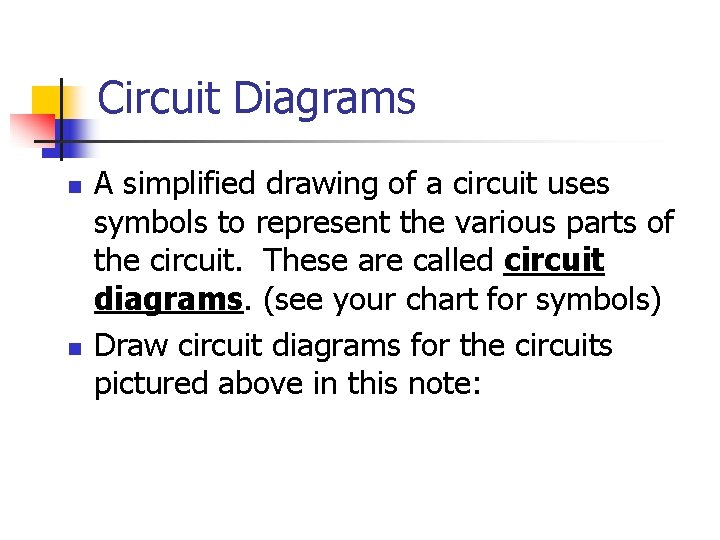Circuit Diagrams n n A simplified drawing of a circuit uses symbols to represent the various parts of the circuit. These are called circuit diagrams. (see your chart for symbols) Draw circuit diagrams for the circuits pictured above in this note:Circuit a) * This is an incomplete circuit since it does not have a load. * This also makes it a short circuit. * However it is a controlled circuit because it contains a switch (open). Dangerous circuit if switch is closed.Circuit b) • This is a complete circuit. • This circuit is controlled because it has a switch (closed)Circuit c) * This is an incomplete circuit since it does not have a load. * This also makes it a short circuit. * This is an uncontrolled circuit because it does not include a switch. * Very dangerous type of circuit.HOMEWORK n n n 1. Read Section 12. 2 in the textbook. Pages have been uploaded to the website. 2. Complete the last two pages of the note (circuit characteristics and drawing circuits) 3. Upload the work to a Google Form Here